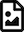# Introduction to Deep Learning (III)

Introduction to Deep Learning (III)

Usage of deep learning algorithm is one of the demanding skills needed in this decade and the coming decade. Providing a hands on experience in using deep learning applications is one of the main goals of this lecture series. Let’s get started.

## Deep Learning Algorithm Part 1

In this part of the lecture series, the idea is to provide an understanding on the usage of various deep learning algorithms. In this lesson, we talk about different algorithms in Deep Learning world. In this lesson we discuss a multi-layer perceptron and convolutional neural networks. Here we use MNIST classification problem and solve it using MLP and CNN.Slides

## Deep Learning Algorithms Part 2

In this lesson, we continue our study on a deep learning algorithms. We use Recurrent Neural Network related examples to show case how it can be applied to do MNIST classfication. We showcase how RNN can be applied to solve this problem.Slides

## Deep Learning Algorithms Part 3

CNN is one of the most prominent algorithms that has been used in the deep learning world in the last decade. A lots of applications has been done using CNN. Most of these applications deal with images, videos, etc. In this lesson we continue the lesson on convolution neural networks. Here we discuss a brief history on CNN.Slides

## Deep Learning Algorithms Part 4

In this lesson we continue our study on CNN by understanding how historical findings supported the upliftment of the Convolutional Neural Networks. And also we discuss why CNN has been used for various applications in various fields.Slides

## Deep Learning Algorithms Part 5

In this lesson we discuss about auto-encoders. This is one of the highly used deep learning based models in signal denoising, image denoising. Here we portray how an auto-encoder can be used to do such tasks.Slides

## Deep Learning Algorithms Part 6

In this lesson we discuss one of the most famous deep neural network architecture, Generative Adversarial Networks. This deep learning model has the capability of generating new outputs from existing knowledge. A GAN model is more like a counter-fitter who is trying to improve itself to generate best counterfits.SlidesSlides